### ROUNDDOWN Function – Examples in Excel, VBA, & Google Sheets

This tutorial demonstrates how to use the Excel ROUNDDOWN Function in Excel to round a number down.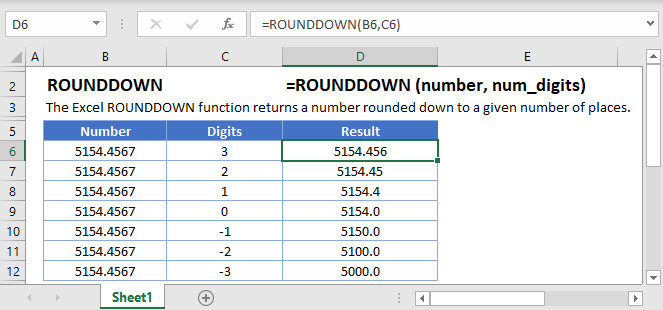## ROUNDDOWN Function Overview

The ROUNDDOWN Function Rounds a number down (towards zero) to a specified number of digits.

To use the ROUNDDOWN Excel Worksheet Function, select a cell and type: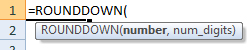(Notice how the formula inputs appear)

### ROUNDDOWN Function Syntax and Inputs:

`=ROUNDDOWN(number,num_digits)`

number – A number.

num_digits – The number of decimal places to round. Negative rounds to the left of the decimal.

AutoMacro - VBA Code Generator

## How to use the ROUNDDOWN Function

### Round down to Nearest Whole Number

To round down to the nearest whole number set the num_digits argument to 0.

`=ROUNDDOWN(A2,0)`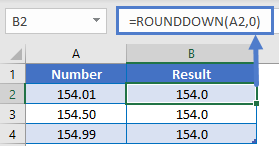### Round down to Two Decimal Places

To round down to two decimal places, set the num_digits argument to 2. Here we will round down a price to the nearest cent:

`=ROUNDDOWN(A2,2)`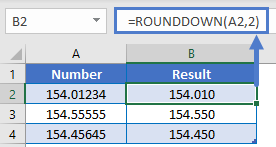### Round down to Nearest 10, 100, or 1000

To round down to the other side of the decimal (to 10, 100, or 1000s), use negative numbers for num_digits:

```=ROUNDDOWN(A2,-1)
=ROUNDDOWN(A2,-2)
=ROUNDDOWN(A2,-3)```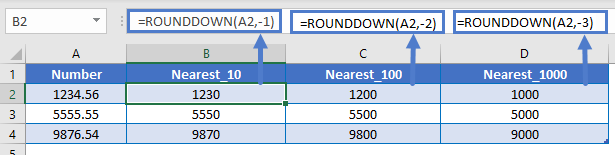### Round down Percentages

When rounding down percentages remember that percentages are decimal values. So to round down to the nearest whole percentage use the ROUNDDOWN Function with num_digits = 2.

`=ROUNDDOWN(A2,2)`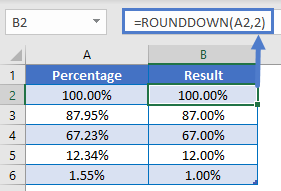## Other ROUND Functions / Formulas

Excel / Google Sheets contains many other round functions. Here are quick examples of each: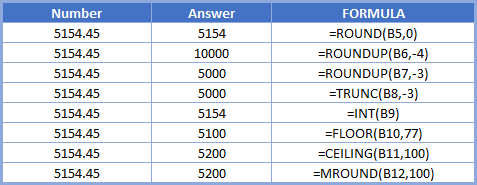Below, we will point out a few functions in particular.

### ROUND and ROUNDUP

The ROUND and ROUNDUP Functions work exactly the same way as the ROUNDDOWN Function, except the ROUND Function uses normal rounding rules and ROUNDUP always rounds up.

```=ROUND(A2,-2)
=ROUNDUP(A2,-2)```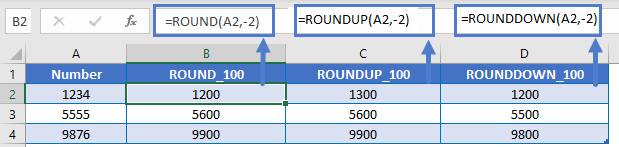### ROUND down to a Multiple

The FLOOR Function is similar to the ROUNDDOWN Function. It will round a number down, but the FLOOR Function allows you to round down to a specific multiple (instead of rounding to a specific number of digits).

`=FLOOR(A2,B2)`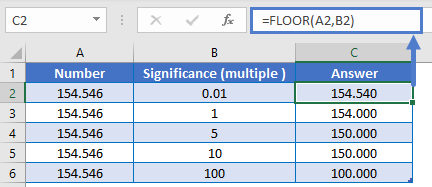The ROUNDDOWN Function works exactly the same in Google Sheets as in Excel: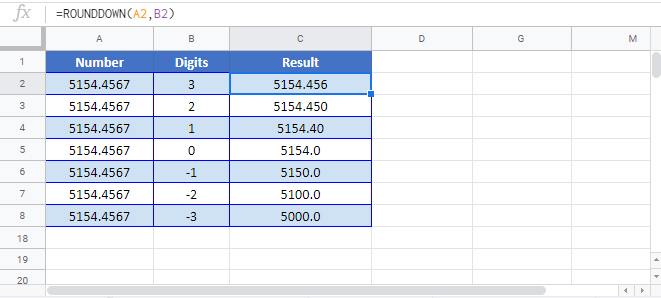## ROUNDDOWN Examples in VBA

You can also use the ROUNDDOWN function in VBA. Type:` application.worksheetfunction.rounddown(number,num_digits)`

Executing the following VBA statements

```Range("C2") = Application.WorksheetFunction.RoundDown(Range("A2"), Range("B2"))
Range("C3") = Application.WorksheetFunction.RoundDown(Range("A3"), Range("B3"))
Range("C4") = Application.WorksheetFunction.RoundDown(Range("A4"), Range("B4"))
Range("C5") = Application.WorksheetFunction.RoundDown(Range("A5"), Range("B5"))
Range("C6") = Application.WorksheetFunction.RoundDown(Range("A6"), Range("B6"))```

will produce the following results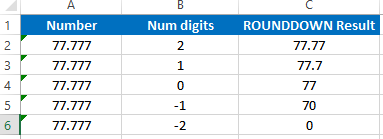For the function arguments (number, etc.), you can either enter them directly into the function, or define variables to use instead.